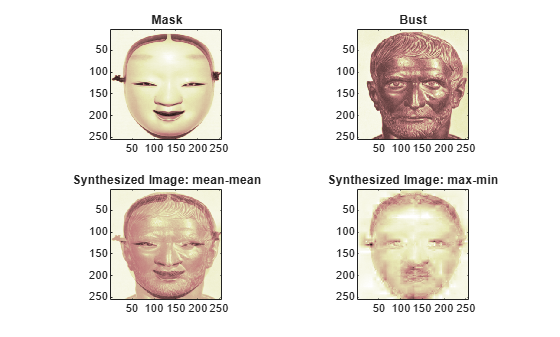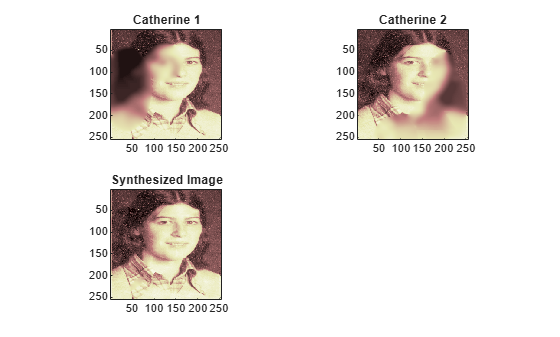## Image Fusion

The toolbox requires only one function for image fusion: `wfusimg`. You'll find full information about this function in its reference page. For more details on fusion methods see the `wfusmat` function.

In this section, you will learn how to:

• Merge images from their decompositions

• Restore images from their decompositions

The principle of image fusion using wavelets is to merge the wavelet decompositions of the two original images using fusion methods applied to approximations coefficients and details coefficients (see [MisMOP03] and [Zee98] in References).

The two images must be of the same size and are supposed to be associated with indexed images on a common colormap (see `wextend` to resize images).

Two examples are examined: the first one merges two different images leading to a new image and the second restores an image from two fuzzy versions of an original image.

### Fuse Two Images

This example shows how to fuse two images to create a new image.

```load mask x1 = X; load bust x2 = X;```

Merge the two images from level 5 wavelet decompositions using the `db2` wavelet. Perform the fusion by taking the mean for both approximations and details.

```wv = 'db2'; lv = 5; xfusmean = wfusimg(x1,x2,wv,lv,'mean','mean');```

Merge the two images again, but this time perform the fusion by taking the maximum of the approximations and the minimum for the details.

`xfusmaxmin = wfusimg(x1,x2,wv,lv,'max','min');`

Plot the original and fused images.

```subplot(2,2,1) image(x1) axis square title('Mask') subplot(2,2,2) image(x2) axis square title('Bust') subplot(2,2,3) image(xfusmean) axis square title('Synthesized Image: mean-mean') subplot(2,2,4) image(xfusmaxmin) axis square title('Synthesized Image: max-min') colormap(map)```### Restore Image From Two Fuzzy Versions

This example shows how to restore an image from two fuzzy versions of an original image.

Load two fuzzy versions of an original image.

```load cathe_1 x1 = X; load cathe_2 x2 = X;```

Merge the two images from level 5 wavelet decompositions using the `smy4` wavelet. Perform the fusion by taking the maximum of the absolute value of the coefficients for both approximations and details.

```wv = 'sym4'; lv = 5; xfus = wfusimg(x1,x2,wv,lv,'max','max');```

Plot the original and fused images.

```subplot(2,2,1) image(x1) axis square title('Catherine 1') subplot(2,2,2) image(x2) axis square title('Catherine 2') subplot(2,2,3) image(xfus) axis square title('Synthesized Image') colormap(map)```